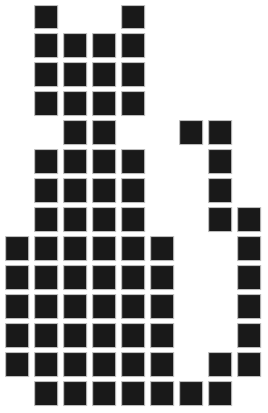# Vectorization with square point buffers

This year (2014) the buffer algorithm of Boost.Geometry was finally released in Boost 1.56

With buffer we mean a zone around a geometry. This is the GIS term, also used by OGC. In other environments it is often known as inflate / deflate, or shrink and expand, or Minkowski sum.

In GIS the buffer (with a positive distance) creates a larger version of e.g. a polygon. If a negative distance is specified, a smaller version is created.

As the documentation shows, various strategies can be used to:
• control the buffer-distance (either symmetric or asymmetric)
• control the joins (rounded or miter)
• control the ends for linestrings (rounded or flat)
• control the sides (straight)
• control the zones around points (or degenerated linestrings) (circular or square)
Strategies are provided, but they can also be provided by the library user, e.g. to create some fancy effects.

This short blog gives an example of the strategies for zones around points, using the square version. This strategy has the nice property that can be used to convert grids or images to vectors (polygons).

## strategy::buffer::point_square

See also the Wiki page. Pixels are converted to polygons. Suppose we add all centers of all filled pixels (e.g. the black pixels) into a multi point. We then buffer that multi-point with a distance of half the pixel size. And we use the strategy to create square buffers around each point. Then all adjacent gridcells will be connected. A polygon (or a multi-polygon) will be created, which is exactly what we wanted...

Suppose we have the following image (from this source) of a black cat.We create a multi-point of it, that is done manually:

```MULTIPOINT(1 0,2 0,3 0,4 0,5 0,6 0,7 0,   0 1,1 1,2 1,3 1,4 1,5 1,7 1,8 1,
0 2,1 2,2 2,3 2,4 2,5 2,8 2, 0 3,1 3,2 3,3 3,4 3,5 3,8 3,   0 4,1
4,2 4,3 4,4 4,5 4,8 4, 0 5,1 5,2 5,3 5,4 5,5 5,8 5,   1 6,2 6,3 6,4
6,7 6,8 6,   1 7,2 7,3 7,4 7,7 7, 1 8,2 8,3 8,4 8,7 8,   2 9,3
9,   6 9,7 9,   1 10,2 10,3 10,4 10,   1 11,2 11,3 11,4
11,   1 12,2 12,3 12,4 12,   1 13,4 13)```

If we use the next code fragment:

`    typedef boost::geometry::model::d2::point_xy<double> point;`
`    typedef boost::geometry::model::polygon<point> polygon;`
`    boost::geometry::strategy::buffer::distance_symmetric<double> distance_strategy(0.5);`
`    boost::geometry::strategy::buffer::join_round join_strategy;`
`    boost::geometry::strategy::buffer::end_round end_strategy;`
`    boost::geometry::strategy::buffer::point_square point_strategy;`
`    boost::geometry::strategy::buffer::side_straight side_strategy;`
`    boost::geometry::model::multi_polygon<polygon> result;`
`    boost::geometry::model::multi_point<point> mp;`
`    boost::geometry::read_wkt(cat, mp); // the cat std::string is somewhere defined`
`    boost::geometry::buffer(mp, result,`
`                distance_strategy, side_strategy,`
`                join_strategy, end_strategy, point_strategy);`

The resulting polygon will look like this: